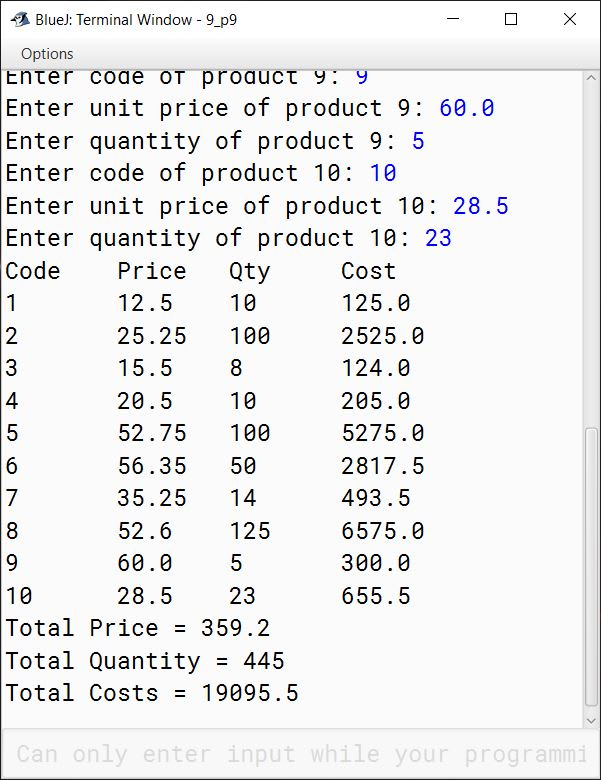# Solved Sample Paper 2

## Section A

#### Question 1

(a) Define Polymorphism.

In object-oriented programming, Polymorphism provides the means to perform a single action in multiple different ways. Taking the real world example of animals, if we ask different animals to speak, they respond in their own way. Dog barks, duck quacks, cat says meow and so on. So the same action of speaking is performed in different ways by different animals.

(b) Differentiate between static variable and instance variable.

Static VariableInstance Variable
Static Variables are declared using keyword 'static'.Instance Variables are declared without using keyword 'static'.
All objects of a class share the same copy of static variables.Each object of the class gets its own copy of instance variables.
Static Variables can be accessed using the class name or object.Instance Variables can be accessed only through an object of the class.

(c) What are identifiers in Java? List three identifier formation rules.

Identifiers are used to name different parts of a program such as variables, methods, classes, objects, etc. Three identifier formation rules are:

1. An an identifier can be a sequence of alphabets, digits, underscore and dollar sign characters only.
2. Identifiers cannot start with a digit.
3. An identifier must not be a Keyword or a Boolean or null literal.

(d) Identify the literals listed below:

1. true
2. 'X'
3. "false"
4. 25.75

1. boolean literal
2. Character literal
3. String literal
4. Floating Point Literal

(e) Name the wrapper classes of char type and long type

Wrapper class of char is Character and long is Long.

#### Question 2

(a) Name the type of error in each case given below:

1. Multiplication operator used instead of division operator
2. Missing parenthesis

1. Logical Error
2. Syntax Error

(b) Write two differences between if-else-if and switch-case statements.

Two differences between if-else-if and switch-case statements are:

1. switch-case statement can only test for equality whereas if-else-if can test for any Boolean expression.
2. switch-case tests the same expression against constant values while if-else-if can use different expression involving unrelated variables.

(c) What is the use of break statement in Java?

The break statement terminates the current loop or switch statement. The execution then continues from the statement immediately following the current loop or switch statement.

(d) Write the prototype of a function check which takes a String and a character as an argument and returns a boolean.

boolean check(String str, char ch)

(e) What is the use of the keyword 'this' in Java programming?

The 'this' keyword refers to the current object in a function or constructor. It is primarily used to resolve the conflict between method parameters and instance variables/fields.

#### Question 3

(a) Write a Java expression for the following:

$\sqrt{\dfrac{(x - h)^2}{a} + \dfrac{(y - k)^2}{b}}$

Math.sqrt((Math.pow((x-h), 2) / a) + Math.pow((y-k), 2) / b)

(b) Give the output of the following:

1. Math.max(Math.ceil(14.5), 15.5)
2. Math.sqrt(Math.abs(-225))

1. 15.5
2. 15.0
##### Explanation
1. Math.ceil(14.5) return 15.0. Replacing return value of Math.ceil in Math.max ⇒ Math.max(15.0, 15.5). 15.5 is the greater of the two hence it is the output.
2. Math.abs(-225) gives 225. Square root of 225 is 15.0.

(c) Evaluate the following if the value of m = 10 and n = 6

m -= 9 % ++n + ++n / 2

m -= 9 % ++n + ++n / 2
⇒ m = m - (9 % ++n + ++n / 2)
⇒ m = 10 - (9 % 7 + 8 / 2)
⇒ m = 10 - (2 + 4)
⇒ m = 10 - 6
⇒ m = 4

(d) Rewrite the following program segment using if-else:

tax = income > 1000000 ? income * 30.0 / 100 : income * 10.0 / 100;

if (income > 1000000)
tax = income * 30.0 / 100;
else
tax = income * 10.0 / 100;

(e) Give the output of the following program segment:

int n[] ={1,2,3,5,7,9,13,16};
double a=Math.pow(n, n);
double b=Math.sqrt(n+n);
System.out.println("a=" + a);
System.out.println("b=" + b);

##### Output
a=49.0
b=5.0

##### Explanation

n is 7 and n is 2. Math.pow(n, n) becomes Math.pow(7, 2) so a becomes 49.0. Next, n is 9 and n is 16. Math.sqrt(n+n) becomes Math.sqrt(9 + 16) ⇒ Math.sqrt(25) ⇒ 5.0

(f) Rewrite the following program segment using the corresponding while loop:

int f=1, i;
for(i=1; i<=5; i++)
f*=i;
System.out.println(f);

int f=1, i=1;
while (i <= 5) {
f*=i;
i++;
}
System.out.println(f);

(g) Give the output of the following program segment:

String a="10", b="20";
int x=Integer.parseInt(a);
int y=Integer.valueOf(b);
System.out.println(x+y);
System.out.println(a+b);

##### Output
30
1020

##### Explanation

Integer.parseInt and Integer.valueOf convert the strings a and b into their corresponding numeric integers. x + y adds 10 and 20 to give 30 as the output. a and b are strings so addition operator concatenates them and prints 1020.

(h) Give the output of the following program:

public class StringCheck {

boolean foo(String str) {
int x = str.compareTo("INCOMMUTABLE");
System.out.println("x=" + x);
boolean ret = x == 0 ? true : false;
return ret;
}

public static void main(String[] args) {
StringCheck obj = new StringCheck();
boolean a = obj.foo("INCOMPUTABLE");
System.out.println(a);
}
}

##### Output
x=3
false

##### Explanation

Output of "INCOMPUTABLE".compareTo("INCOMMUTABLE") will be:

ASCII of P - ASCII of M
⇒ 80 - 77
⇒ 3

Value of x becomes 3. As x is not equal to 0 so ternary operator assigns false to ret. foo method returns back this false that gets assigned to a in main method and then printed as the output.

(i) State a difference between constructor and function.

Constructor has the same name as class name whereas function should have a different name than class name.

(j) For a class Toy, create two objects "Barbie" and "Stack"?

Toy Barbie = new Toy();
Toy Stack = new Toy();

## Section B

#### Question 4

Define a class named Pay with the following description:

Instance variables /Data members:

String name — To store the name of the employee

double salary — To store the salary of the employee

double da — To store Dearness Allowance

double hra — To store House Rent Allowance

double pf — To store Provident Fund

double grossSal — To store Gross Salary

double netSal — To store Net Salary

Member methods:

Pay(String n, double s) — Parameterized constructor to initialize the data members.

void calculate() — To calculate the following salary components:

1. Dearness Allowance = 15% of salary
2. House Rent Allowance = 10% of salary
3. Provident Fund = 12% of salary
4. Gross Salary = Salary + Dearness Allowance + House Rent Allowance
5. Net Salary = Gross Salary - Provident Fund

void display() — To display the employee name, salary and all salary components.

Write a main method to create object of the class and call the member methods.

import java.util.Scanner;

public class Pay
{
String name;
double salary;
double da;
double hra;
double pf;
double grossSal;
double netSal;

public Pay(String n, double s) {
name = n;
salary = s;
da = 0;
hra = 0;
pf = 0;
grossSal = 0;
netSal = 0;
}

void calculate() {
da = salary * 15.0 / 100;
hra = salary * 10.0 / 100;
pf = salary * 12.0 / 100;
grossSal = salary + da + hra;
netSal = grossSal - pf;
}

void display() {
System.out.println("Employee Name: " + name);
System.out.println("Salary: " + salary);
System.out.println("Dearness Allowance: " + da);
System.out.println("House Rent Allowance: " + hra);
System.out.println("Provident Fund: " + pf);
System.out.println("Gross Salary: " + grossSal);
System.out.println("Net Salary: " + netSal);
}

public static void main(String args[]) {
Scanner in = new Scanner(System.in);
System.out.print("Enter Employee Name: ");
String empName = in.nextLine();
System.out.print("Enter Salary: ");
double empSal = in.nextDouble();

Pay obj = new Pay(empName, empSal);
obj.calculate();
obj.display();
}
}
##### Output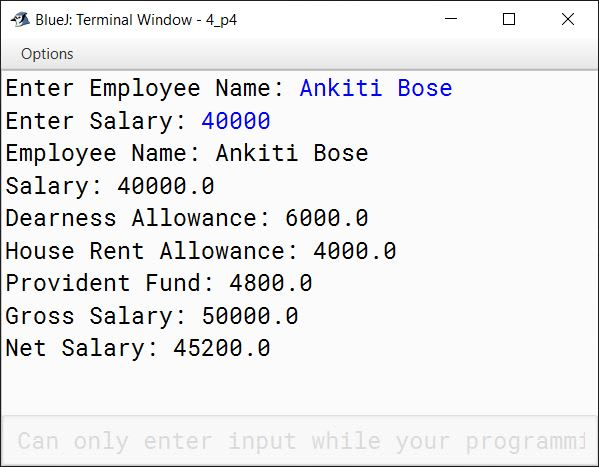#### Question 5

A Dudeney number is a positive integer that is a perfect cube such that the sum of its digits is equal to the cube root of the number. Write a program to input a number and check and print whether it is a Dudeney number or not.

Example:

Consider the number 512.

Sum of digits = 5 + 1 + 2 = 8
Cube root of 512 = 8

As Sum of digits = Cube root of Number hence 512 is a Dudeney number.

import java.util.Scanner;

public class KboatDudeneyNumber
{
public static void main(String args[]) {
Scanner in = new Scanner(System.in);
System.out.print("Enter the number: ");
int n = in.nextInt();

//Check if n is a perfect cube
int cubeRoot = (int)Math.round(Math.cbrt(n));
if (cubeRoot * cubeRoot * cubeRoot == n) {
//If n is perfect cube then find
//sum of its digits
int s = 0;
int t = n;
while (t != 0) {
int d = t % 10;
s += d;
t /= 10;
}

if (s == cubeRoot) {
System.out.println(n + " is a Dudeney number");
}
else {
System.out.println(n + " is not a Dudeney number");
}
}
else {
System.out.println(n + " is not a Dudeney number");
}
}
}
##### Output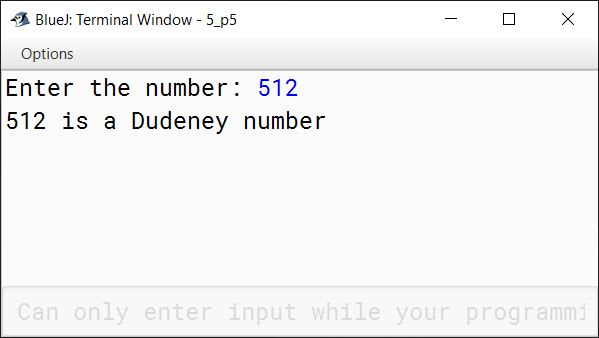#### Question 6

Write a menu-driven program to display the following patterns as per the user’s choice:

(a)

1234567
12345
123
1

(b)

11111
22222
33333
44444
55555

import java.util.Scanner;

public class KboatPatterns
{
public static void main(String args[]) {
Scanner in = new Scanner(System.in);
System.out.println("Type 1 for Pattern 1");
System.out.println("Type 2 for Pattern 2");
System.out.print("Enter your choice: ");
int ch = in.nextInt();
switch (ch) {
case 1:
for (int i = 7; i >= 1; i -= 2) {
for (int j = 1; j <= i; j++) {
System.out.print(j);
}
System.out.println();
}
break;

case 2:
for (int i = 1; i <= 5; i++) {
for (int j = 1; j <= 5; j++) {
System.out.print(i);
}
System.out.println();
}
break;

default:
System.out.println("Incorrect Choice");
}
}
}
##### Output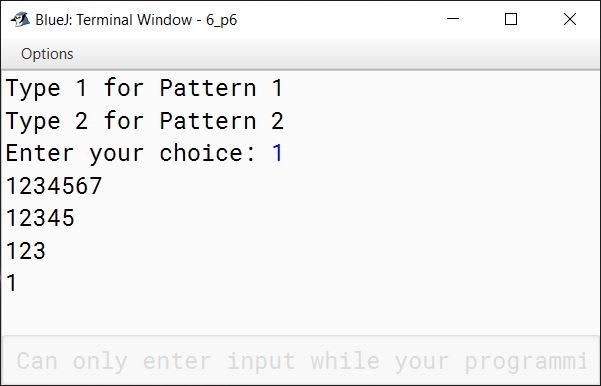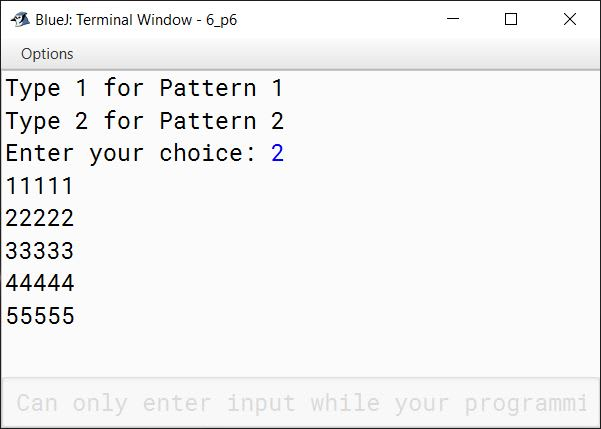#### Question 7

Design a class to overload a function series( ) as follows:

(a) void series(int a, int n) — To display the sum of the series given below:

a - (a/2!) + (a/3!) - (a/4!) + ...... n terms

(b) void series(int n) — To display the sum of the series given below:

1/2 - 2/3 + 3/4 - 4/5 + ...... n terms

Write a main method to create an object and invoke the above methods.

import java.util.Scanner;

{
void series(int a, int n) {
double sum = 0;
int m = 1;
for (int i = 1; i <= n; i++) {
long f = 1;
for (int j = 1; j <= i; j++) {
f *= j;
}
double t = (double)a / f * m;
sum += t;
m *= -1;
}
System.out.println("Series Sum = " + sum);
}

void series(int n) {
double sum = 0;
int m = 1;
for (int i = 1; i <= n; i++) {
double t = ((double)i / (i + 1)) * m;
sum += t;
m *= -1;
}
System.out.println("Series Sum = " + sum);
}

public static void main(String args[]) {
Scanner in = new Scanner(System.in);
System.out.print("Enter a: ");
int a = in.nextInt();
System.out.print("Enter n: ");
int n = in.nextInt();

obj.series(a, n);
obj.series(n);
}
}
##### Output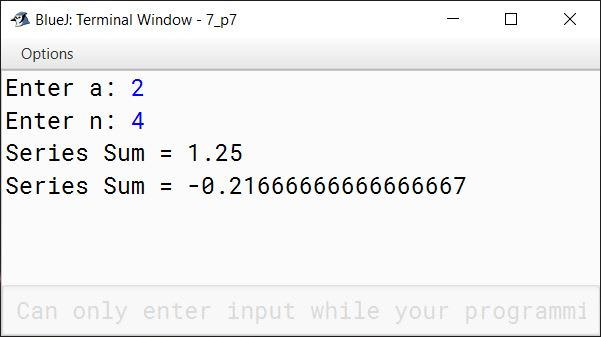#### Question 8

Write a program to input 10 alphabets in an array. Now ask the user to input an alphabet and search if the alphabet is present in the array or not using linear search technique. If found display "Search Successful" and print the index of the alphabet in the array, otherwise display "Search Unsuccessful".

import java.util.Scanner;

public class KboatLinearSearch
{
public static void main(String args[]) {
Scanner in = new Scanner(System.in);
char arr[] = new char;
System.out.println("Enter 10 alphabets:");
for (int i = 0; i < 10; i++) {
arr[i] = in.next().charAt(0);
}

System.out.print("Enter alphabet to search: ");
char ch = in.next().charAt(0);

int i = 0;
for (i = 0; i < 10; i++) {
if (arr[i] == ch) {
break;
}
}

if (i == 10) {
System.out.println("Search Unsuccessful");
}
else {
System.out.println("Search Successful");
System.out.println(ch + " present at index " + i);
}
}
}
##### Output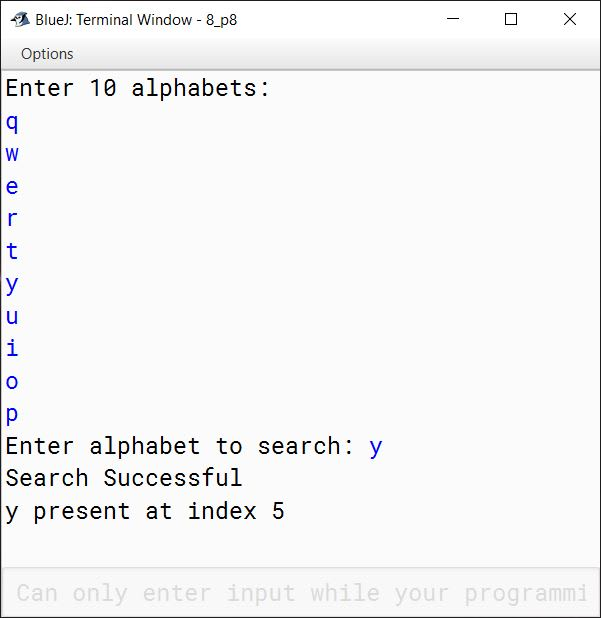#### Question 9

Write a program to create three single dimensional arrays cod[], pric[], qty[] to store product code, unit price and quantity of each product for 10 products. Calculate the total cost of each product and print the result in tabular form including product code, unit price, quantity and total cost of each product. At the end print total of price, total of quantity and the total of costs.

import java.util.Scanner;

public class KboatProducts
{
public static void main(String args[]) {
Scanner in = new Scanner(System.in);
int cod[] = new int;
double pric[] = new double;
int qty[] = new int;

for (int i = 0; i < 10; i++) {
System.out.print("Enter code of product " + (i + 1) + ": ");
cod[i] = in.nextInt();
System.out.print("Enter unit price of product " + (i + 1) + ": ");
pric[i] = in.nextDouble();
System.out.print("Enter quantity of product " + (i + 1) + ": ");
qty[i] = in.nextInt();
}

double tp = 0;
long tq = 0;
double tc = 0;

System.out.println("Code\tPrice\tQty\tCost");
for (int i = 0; i < 10; i++) {
double cost = qty[i] * pric[i];
tp += pric[i];
tq += qty[i];
tc += cost;
System.out.println(cod[i] + "\t" + pric[i] + "\t" + qty[i] + "\t" + cost);
}

System.out.println("Total Price = " + tp);
System.out.println("Total Quantity = " + tq);
System.out.println("Total Costs = " + tc);
}
}
##### Output